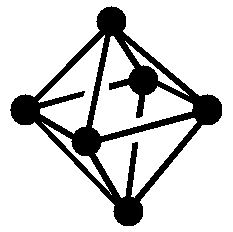Name: ______________________________

Section: _____________________________

Student ID#:__________________________

Work in groups on these problems. You should try to answer the questions without referring to your textbook. If you get stuck, try asking another group for help.

Learning Objectives

• Understand that skeletal electron count effects the geometry of polyhedral clusters
• Be able to predict the structures of different clusters based on molecular formulae
• Be able to determine how many electrons a group might contribute to a cluster

The element Boron is only one element away from Carbon, often overlooked, its chemistry in many ways is as rich and diverse as its ubiquitous neighbor. You might remember from general chemistry, when writing the Lewis Dot Structures for boron compounds, it was frequently in environments that violated the octet rule. This tendency toward electron deficient bonding (preferring to have less than 8 electrons in its bonding environments) has significant consequences regarding the structures and types of molecules boron forms. Today’s focus will be the structural diversity of the boron hydrides and how we predict structure based on electronics. One way of predicting the structure of these complex, three dimensional molecules is to employ Wade’s Rules. Wade’s Rules are an electron counting method for dealing with these electron deficient and structurally complex molecules. The figures below are visual representations of these rules, use them to guide you through the Key Questions.Figure 1: Skeletal Electrons are the free valence electrons donated by the atoms or atom units that comprise the cluster.

 Structure (• = BH) Note: Protons omitted from drawings for clarityMolecular Formula B6H62- B5H9 B4H10 Name of Structure closo nido arachno Equations for calculating skeletal electron count Note: n is the number of vertices or non-H atoms 2n + 2 2n + 4 2n + 6 Number of skeletal electrons 14 14 14

## Key Questions

1. Progressing from left to right on Figure 2, each structure is based on the first closo (closed) structure. How many vertices are removed from the closo structure to form the arachno structure?
2. What is another name for skeletal electrons?
3. How many skeletal electrons are required for an arachno structure that has 10 vertices?
4. Predict a structure.
1. How many BH units are there in B10H14?
2. How many leftover H’s (Hydrogens not part of BH units) are there in B10H14?
3. How many skeletal electrons are in the cluster?
4. What is the value of n?
5. What would be the predicted structure for B10H14?
5. How do you incorporate the charge on B6H62- into your skeletal electron count?
6. Referring to Figure 1, how many skeletal electrons will a CH unit contribute to the skeletal electron count? Show pictorially how you arrive at this conclusion.
7. Predict the structure of the compound C2B4H6. (Hint use the leading questions from #4.)
9. In your own words, write a stepwise procedure for using Wade’s Rules to predict a structure.

## Exercises

1. Using Wade’s Rules, predict the structure for C2B10H12.
1. How many electrons would a Cl-Mo fragment contribute to a cluster’s skeletal electron count?
2. What is the skeletal electron count for the cluster, Mo6Cl84+?
3. Is this cluster closo-, nido-, arachno-?
4. Based on this information, draw the structure for Mo6Cl84+.

## Reference

• Kelley J. Donaghy, SUNY-ESF